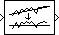# Detrend

Remove linear trend from vectors

## Library

Statistics

`dspstat3`

•## Description

The Detrend block removes a linear trend from the length-M input vector, u, by subtracting the straight line that best fits the data in the least squares sense.

The least squares line, û = ax + b, is the line with parameters a and b that minimizes the quantity

`$\sum _{i=1}^{M}{\left({u}_{i}-{\stackrel{^}{u}}_{i}\right)}^{2}$`

for M evenly-spaced values of x, where ui is the ith element in the input vector. The output, y = u-û, is always an M-by-1 column vector.

## Supported Data Types

• Double-precision floating point

• Single-precision floating point

 Cumulative Sum DSP System Toolbox Difference DSP System Toolbox Least Squares Polynomial Fit DSP System Toolbox Unwrap DSP System Toolbox `detrend` MATLAB